Next Article in Journal
Elementary Iodine-Doped Activated Carbon as an Oxidizing Agent for the Treatment of Arsenic-Enriched Drinking Water
Next Article in Special Issue
Physical Modelling of Arctic Coastlines—Progress and Limitations
Previous Article in Journal
The Effect of Modifying a CFD-AB Approach on Fish Passage through a Model Hydraulic Dam
Open AccessArticle

# Numerical Simulation of the Sound Field of a Five-Stage Centrifugal Pump with Different Turbulence Models

1
National Research Center of Pumps, Jiangsu University, Zhenjiang 212013, China
2
National Engineering and Technology Center for Information Agriculture, Nanjing 210095, China
*
Authors to whom correspondence should be addressed.
Water 2019, 11(9), 1777; https://doi.org/10.3390/w11091777
Received: 28 June 2019 / Revised: 22 August 2019 / Accepted: 23 August 2019 / Published: 26 August 2019
(This article belongs to the Special Issue Innovative Model Strategies in Hydraulics)

## Abstract

To study the influence of the turbulence model on the sound field of pumps, the standard k-ε, Re-normalization Group (RNG) k-ε and Shear Stress Transfer (SST) k-ω models were employed to simulate flow and sound fields of a five-stage centrifugal pump with a vaned-diffuser. The vibration characteristics of the pump were simulated with the modal response method. A vibration experiment in the pump was carried out to verify the feasibility of the numerical simulation of the hydrodynamic noise in the pump. Results show that in the spectrum of internal and external noise, the peak value appears at axial passing frequency (APF) and its harmonic frequency. Compared with the standard k-ε model, the RNG k-ε and SST k-ω models show good consistence with the noise characteristics of experimental results, indicating the characteristic frequency and revealing the approximate behavior of the sound field in the pump. In general, the simulation of the sound field based on the RNG k-ε model is most appropriate for the multistage centrifugal pump with a vaned-diffuser.

## 1. Introduction

The multistage centrifugal pump is a fundamental equipment used in the process industry. Because of its high efficiency, wide performance and stable operation, it has been widely utilized in refineries, power plants, and so forth . Nowadays the problem of excessive vibration and noise has become a rising concern [2,3,4,5]. In the internal flow field of centrifugal pumps, a rotating impeller drives fluid flow, the load function of the fluid causes structure vibration in the flow process, and then ultimately produces radiated noise. Meanwhile, the flow in the channel of the centrifugal pump is very complicated, and involves unsteady turbulence, such as secondary flow, gap flow, backflow, cavitation, etc. Unsteady flow leads to local pressure fluctuation and then propagates at the acoustic speed, due to the existence of rotor-stator interaction (RSI) , unreasonable structure design, etc. The unsteady flow field induces a complicated force pattern on the structural components and further leads to superlative vibration and noise. Flow field and noise experiments of multistage centrifugal pumps are expensive and time consuming, therefore, numerical simulations and performance prediction based on computational fluid dynamics (CFD) have been a research focus, which could reduce or even replace some laboratory experiments. In recent years, numerical simulations with the time-averaged Navier–Stokes (N–S) equations are widely used in analysis and optimization of the flow field in pumps [7,8,9,10,11].
The study of induced noise can be traced back to the 1950s, and the purpose was to reduce the noise of submarines as much as possible, which led to the prosperity of acoustics research. So far, there have numerous studies been conducted on the flow induced noise of pumps. Chu et al.  measured the internal fluid field of centrifugal pumps using particle image velocimetry (PIV) and obtained the pressure distribution by simulation. The results show that the main factor that leads to the production of induced noise was the RSI. An experimental study made by Langthjem and Olhof  showed that the influence of the dipole source takes the dominant position in the noise of a centrifugal pump. Chini et al.  investigated the external noise of a pump, and the results indicated that the noise examination could be taken as a valid means to determine whether cavitation occurs. The flow induced noise of a centrifugal pump was studied by Srivastav et al.  to assess the influence of the clearance between impeller and volute on the radiated noise. They found that noise decreases with increasing clearance.
Regarding numerical simulations, it is essential to choose the most appropriate turbulence model. Ji et al.  modified a partially-averaged N–S computational model to handle both cavitation and turbulence. In a centrifugal pump with short length blades, Thai and Lee  applied a standard two-equation model coupled with a homogeneous mass transfer model to simulate the cavitation behavior and drew the conclusion that additional half-length blades can eliminate cavitation and induced pressure oscillations well. Zhang et al.  aimed to simulate and analyze the tip-leakage flow structure and instantaneous evolution of tip vortex cavitation in a scaled axial-flow pump. In recent years, a filter-based model (FBM) is gradually applied to the simulation of turbulent cavitating flows. In several hydrofoils, the application of Reynolds-averaged Navier–Stokes (RANS) equations and FBM in unsteady cavitation flow is compared [19,20,21,22]. Later on, an improved FBM with the density correction method was proposed by Wang et al. , and its superiority over the FBM was also highlighted. Considering that the multi-stage pump deals with high-heads, experimental results are highly affected by the accuracy of the experiment bench and installation debugging. It is necessary to choose a reliable turbulence model for appropriate numerical simulations.
Thus, a five-stage centrifugal pump with a vaned-diffuser is investigated herein and new laboratory results were chosen as reference to verify the feasibility of the sound field calculation. Based on the software of ANSYS-CFX and the Siemens LMS Virtual lab, the prediction accuracy of the pump under different flow rates was compared by choosing different turbulence models, to provide the basis for an accurate performance and noise prediction of multi-stage centrifugal pumps, and further lay the foundation for design and optimization.

## 2. The Laboratory Model

A five-stage centrifugal pump with a vaned-diffuser, as shown in Figure 1, was used as a laboratory model. Table 1 provides the main structure parameters of the pump. To meet the needs of the head and cavitation performance, the number of blades of the first-stage impeller is six, and for the subsequent four-stage impellers it is seven. The design flow rate Qd is 100 m3/h, the head is 165 m, and the rated rotational speed is 1480 r/min. The main flow passage components are shown in Figure 2.

## 3. Numerical Simulation of Energy Performance

#### 3.1. Mesh Generation and Boundary Conditions

The whole flow field of the five-stage centrifugal pump with a vaned-diffuser includes the suction section, the discharge section, and the water body of all five-stage impellers and diffusers, as shown in Figure 3. The hexahedral structured grid were built by the ANSYS-ICEM software.
Theoretically, the error caused by the grid resolution decreases or even disappears when the grid number increases. However, the number of grid points can not be excessive considering the computational cost. It is therefore necessary to perform a convergence test. Figure 4 shows the convergence test and the trend of the mesh error of the mesh scheme. Divided into seven grid schemes according to the density of the mesh grid and by adopting the time-averaged N–S equations, a numerical simulation under the design flow rate was carried out based on the Re-normalization Group (RNG) k-ε model. Comparing the head prediction value of seven schemes, the results show that scheme A, B, and C may lead to a high error of head prediction, scheme D is suitable as its head deviates from the one from scheme E, F, and G by less than 0.1%. Therefore, scheme D was chosen for the main simulations, which involves 7,803,519 grid points.
In this paper, the standard k-ε, the RNG k-ε and the Shear Stress Transfer (SST) k-ω models were all adopted to simulate the flow field of the five-stage centrifugal pump with a vaned-diffuser under 0.8 Qd, 1.0 Qd, and 1.2 Qd. Multiple coordinate systems were adopted, the impeller field was described with a stationary coordinate system, the other fields were described with a rotating coordinate system. The data transfer surface was used to transfer data between the two coordinate systems. No-slip boundaries were set in the computational domain, and the scalable wall function was used in the near wall. The inlet was set as total pressure and the outlet was set as mass flow rate in the boundary condition, which has a high simulation precision for the pressure fluctuation. The constant time step was 0.002027 s, and the transient time step ΔT was 0.0001126 s which represents the time required for one cycle of impeller rotation. Once apparent periodicity appeared in the flow field and became stable, the pressure pulsation information over eight simulation periods were extracted as source excitation.

#### 3.2. Comparison Energy Characteristic between Experiment and Numerical Simulation

The test system including the pump circulation system and the INV3020C signal acquisition system was employed. The TPA-3 electric measuring instrument was taken as the parameter acquisition system for the external characteristics, and a matching analysis software was used to do a real-time survey on the pressure signal, flow signal, speed signal, current voltage, and power signal to obtain the external characteristic curve of the model pump. Figure 5 shows the test system, and Figure 6 provides the comparison of head and efficiency between the simulations and experiments. Under 0.8 Qd, it was obvious that the head prediction value of all three turbulence models is consistent with the experimental values and differ by 1.7%, and the efficiencies are also larger than the experimental values. At 1.0 Qd, the head values of the three models are most similar to the experimental values, with a deviation of only 1.48%. The efficiency prediction values of the three models are similar, but all are larger than the experimental values. At 1.2 Qd, the error is relatively large. The head deviation of the RNG k-ε model is the smallest, and the head deviation with the standard k-ε model is the largest out of the three models.
In general, the simulation methods meet engineering needs. However, the simulated values are larger than the experimental values, due to neglecting the leakage losses and mechanical losses. Generally speaking, the predicted curve based on the RNG k-ε model shows the best agreement with the experimental values.

#### 3.3. Pressure Fluctuation Analysis

The pressure fluctuation was measured with a high frequency dynamic pressure transmitter HY6503, with a deviation limited to 0.5%. Figure 7 shows the pressure pulsation at the outlet pipe line, at four times the diameter downstream from the outlet of the discharge section. Additionally, the quantitative degree of pressure pulsation was represented by the pressure fluctuation coefficient Cp as:
$C p = p − p ¯ 1 2 ρ U t i p 2$
where p is the instantaneous pressure, $p ¯$ denotes the time-averaged pressure, ρ is the density of water, and Utip denotes the circumferential velocity of tip clearance.
The peak value of pressure pulsation appears at 444 Hz, which represents 18 times the axial passing frequency (APF). This is due to the staggered arrangement of the impeller blades and the difference of the phase angle when the impeller is rotating. The pressure pulsation of the experiment is lower than that of the simulations, and there appears undulation in the high-range frequency especially at 1.2 Qd. A possible explanation is that in the actual experiment, the ambient element could not be filtered entirely and cavitation may occur when the flow rate is enhanced.
At 0.8 Qd, the secondary high frequency of pressure pulsation is seven times larger than the value at APF. The predicted values of pulsation at 148 Hz with the RNG k-ε and SST k-ω models are almost two times larger than for the standard k-ε model. A prominent peak value appears at 666 Hz (27 APF), indicating that the influence of the positive diffuser number on the pressure pulsation cannot be neglected. Instead, because the axial clearance between the negative vaned-diffuser and the later-stage impeller is much larger than between the impeller and the positive vaned-diffuser, and the RSI is small, the influence of the negative vaned-diffuser number is relatively weak.
At 1.0 Qd, the peak values of pressure pulsation at 444 Hz with the RNG k-ε and SST k-ω models are about 0.014, which is 1.5 times larger than with the standard k-ε model. Compared with the other two models, many fluctuations in the curve of the standard k-ε model are observed. The pressure fluctuation at 1.0 Qd is basically the smallest. Once the pump is working at the designed operating point, the streamline and circulation of the fluid field is rather productive and stable.
At 1.2 Qd, the curve based on the standard k-ε model is the smoothest. Additionally, there appear peak values in the high-frequency range, which may indicate that the standard k-ε model is unsuitable for pressure pulsation prediction under large flow rates.

## 4. Further Methodical Details

#### 4.1. Vibration Simulation and Experimental Verification

Due to the non-configured electric motor in the simulation model and the high difficulty of considering background noise precisely, the experimental precision could not be ensured when measuring the noise. Therefore, the simulated and experimentally measured vibration was used to verify the feasibility of noise. Figure 8 shows the experiment and simulation model of vibration.
Under the excitation of the interior fluid force, the damped vibration response equation of centrifugal pumps can be expressed as:
$[ M ] { δ ¨ } + [ C ] { δ ˙ } + [ K ] { δ } = { P ( t ) }$
where [M] is the mass matrix, [C] denotes the damping matrix, [K] is the stiffness matrix, {δ} denotes the displacement vector of the structural node, and {P(t)} represents the loading vector applied to the structure. The damping matrix [C] expresses the linear combination of the mass matrix and stiffness matrix according to Rayleigh theory .
$[ C ] = α [ M ] + β [ K ]$
$α = 2 ( ξ i ω j − ξ j ω i ) ( ω j + ω i ) ( ω j − ω i ) ω i ω j$
$β = 2 ( ξ i ω j − ξ j ω i ) ( ω j + ω i ) ( ω j − ω i )$
where ωi and ωj represent the inherent frequency of order i and j, respectively, and ξi and ξj denote the damping ratio of the modal shape of order i and j. It is hypothesized that ξ = ξi = ξj, the damping ratio is chosen as 0.04 according to the literature [25,26], and then Equations (4) and (5) are written as:
$α = 2 ξ ω i ω j ( ω j + ω i )$
$β = 2 ξ ( ω j + ω i )$
The numerical simulation of vibration was based on the Finite Element Method (FEM). The pump body was made of cast iron, the Young’s modulus E was 135 GPa with density ρ = 7000 kg/m3, and a Poisson ratio μ of 0.3 and a damping ratio of 0.01 were chosen. According to the actual experimental conditions, relevant constraints were exerted on the ground and foot. The velocity along the pipe shaft was constrained at 0, and the foot constraint was set as full-loaded. The loading excitation was the time-averaged transient pressure distribution of the fluid surface and was mapped on the pump structure with interpolation search algorithms, taken as the dynamic load vector on the shell mesh.
The measuring point of the vibration experiment was the same as in the simulation, and the DII301 capacitive acceleration sensor was selected as the vibration sensor. The sampling frequency was set as 5120 Hz which satisfies the Nyquist Sampling equation , and the sampling time was 60 s. The vibration acceleration data was transformed into the vibration velocity data by first-order integral.

#### 4.2. Sound Field

The dipole source plays a dominant role in flow induced noise of centrifugal pumps, whilst the monopole and quadrupole may be neglected . The excitation represented by the wall dipole source was extracted from the transient flow field of the pump, which was necessary for subsequent vibration and noise simulation, and that the source is of consistence. To ensure the accuracy of noise simulation, the sound source included the dipole source in the impellers and vaned-diffusers, as well as the dipole source fixed in the wall. Based on Lighthill theory , the interconnection between fluid field and noise field is written as
$1 c 2 ∂ 2 p ′ ∂ t 2 − ∂ 2 p ′ ∂ x i 2 = ∂ 2 T i j ∂ x i ∂ x j − ∂ ∂ x i ( p i j n j δ ( f ) ) + ∂ ∂ t ( p 0 v j n j δ ( f ) )$
where $p ′ = p − p 0$, which is the fluctuating pressure and equals the sound pressure in the far sound field, c is the sound velocity, t denotes time, xi denotes the space coordinates, vj represents the fluid velocity, nj is the component of the surface normal vector, Tij denotes the Lighthill tensor, and $T i j = ρ v i v j + [ ( p − p 0 ) − c 2 ( ρ − ρ 0 ) ] δ i j − τ i j$; ρ and ρ0 are the fluid density and average density, respectively. τij is the viscous stress tensor, δ(f) is the Dirac function which describes the instant position of the surface, pijnj is the normal force on a unit area on which the objects act on the fluid. $p i j = ( p − p 0 ) δ i j − τ i j$, and δij is the Kronecker symbol. After the fluid properties are resolved for each time step, Equation (8) can be solved for p’, which play a role in the excitation sources.

#### 4.2.1. Internal Sound Field

The pressure pulsation obtained from the CFD results was interpolated over the Boundary Element Method (BEM) acoustic model and was then taken as the boundary condition in the acoustic simulations. The distribution of the dipole source and the boundary conditions solved by Equation (8) is shown in Figure 9. The inlet and outlet of the pump were set as absorption property, the acoustic impedance was 1.5 × 106 kg·m−2·s−1, and the other fluid surfaces were set as total reflection walls. The sound speed in the water was 1500 m/s, and the referenced sound pressure was 1 × 10−6 Pa.
The induced flow noise was measured with an ST60 hydrophone which was installed by a flush type, and the sensor probe was located at the pipe wall. The arrangement method prevented the measuring signal from including the fake sound of the sensor surface produced by turbulent fluctuation pressure.

#### 4.2.2. External Sound Field

Five monitoring points of M1, M2, M3, M4, and M5 in the experiment were located at a distance of 1 m from the pump, and the height from the ground was 1 m as well, as shown in Figure 10.
In the simulations of the external sound field, 36 monitoring points were located at intervals of 10°, and the center was the kernel of the third impeller, as shown in Figure 11. Meanwhile, the A–A horizontal direction and B–B vertical direction are defined in Figure 11. The suction and discharge surfaces are defined as sound absorption and the acoustic impedance was 1.5 × 106 kg·m−2·s−1. The flow passage surface was modelled as vibro-acoustic coupling, and the other surfaces were total-reflection walls. In the external field, the acoustic medium was air, its acoustic impedance was 416.5 kg·m−2·s−1, and the speed of sound was 340 m/s.

## 5. Results and Discussion

#### 5.1. Vibration Analysis

Figure 12 shows the vibration contrast between the simulation and experiment under 1.0 Qd. The APF is 24.67 Hz, the first blade passing frequency (BPF1) is 148 Hz, and the secondary blade passing frequency (BPF2) is 172 Hz. Obvious peak values of vibration appear at the APF, BPF1, and BPF2, and the maximum appears at BPF2. The simulated value at the APF is slightly higher than the experimental value, but it is still in good agreement. The tendency of the vibration simulation is almost consistent with the experimental results, and the deviation is small which implies that the numerical simulation method is feasible. In addition, peak values emerge at BPF1, BPF2, and other frequencies, which could be attributed to the combined interference of the rotor and background noise. On the other hand, the BPF2 of the standard k-ε model is higher than that of the RNG k-ε and SST k-ω models, and the high-frequency band is supposed to be distinct, the reason for this may be the intricate environmental factors. In subsequent research, the reality factor should also be considered.

#### 5.2. Internal Sound Field Analysis

Figure 13 shows the sound pressure level Lp at suction and discharge of the pump which is defined as
$L p = 10 log ∫ f 0 f m a x ( p / 2 ) 2 p r e f 2 d f$
where pref is the referenced sound pressure, which is generally taken as 1 × 10−6 Pa, df is the frequency interval (1.162 Hz), and fmax is the analysis frequency (2000 Hz).
Table 2 shows the deviation of the internal sound field between the turbulence models and the experiments. It can be seen that the experimental values are significantly smaller than the simulated values. The choice of the turbulence model is therefore important. The deviations between the standard k-ε model and the experimental values are the largest with a range of 2.76%~7.35%, and the deviations between the RNG k-ε model and the experiments are the smallest with 0.83%~3.13%. The deviations of Lp at BPF and the harmonic frequency between the RNG k-ε model and the experimental values are the smallest.
In Figure 13, Lp in the suction region stays at 108 dB and at 114 dB in the discharge region. At 1.2 Qd, the deviations within the simulations cannot be neglected. This could be explained by cavitation occurring at suction, where the bubbles begin to collapse and perish under the extrusion force when flowing toward a high-pressure area. Then the center of the bubbles produces high frequency shock waves which induce cavitation noise. The reason why Lp of the SST k-ω model is higher than that of the RNG k-ε model may be that the turbulent kinetic energy of the SST k-ω model is sensitive to free boundary conditions at the exterior margin of the boundary layer and the tiny turbulent frequency ω of the boundary would result in an overestimation of turbulent kinetic energy k.
Figure 14 shows the comparison of the noise spectrum of the five-stage pump at the suction part for the simulations and experiments. It was found that at 0.8 Qd, the peak value of sound pressure level (SPL) appears at BPF1, BPF2, and their harmonic frequency in the spectrum, which attributes to the RSI function. The sound field result based on all three models could be obtained by the variation tendency of noise, and the maximum error relative to the experimental data is less than 5%. At 1.0 Qd, the curve with the RNG k-ε model is most similar to the experiment, because when the pump was working at the design operating point, the flow field of the pump was quite stable and smooth and the flow in the flow passage components is rather uniform. The errors of the standard k-ε, RNG k-ε, and SST k-ω models are all relatively small, however, the curves based on the RNG k-ε and SST k-ω models are closer to the experimental results. At 1.2 Qd, the internal noise enlarges and approaches the maximum which is attributed to the combined action of cavitation and intense pressure pulsation. The most appropriate model is once more the RNG k-ε model.
The large deviation of the results of the standard k-ε model could be explained as follows: in this turbulence model, the dynamic eddy viscosity coefficient μt is assumed to be isotropic scalar, however, it should be an anisotropic tensor in most cases. This leads to certain distortions when applied to a strong swirl. However, a turbulent vortex with a three-dimensional, unsteady and complex property is considered in the RNG k-ε turbulence model [30,31], and the RNG k-ε model involves extra terms to calculate k and ε on the basis of the standard k-ε model, affecting the eddy factor and low-Reynolds action. In contrast, the SST k-ω model takes advantage of the boundary layer under various pressure gradients, and the SST k-ω model employs k-ω pattern in the internal region near the wall and results in higher resolution with more physical significance. In general, the fluid field calculated by the RNG k-ε model is meticulous, and swirling flows are taken into account by modifying the eddy viscosity.
SPL at the discharge part of the pump is shown in Figure 15. The peak values appear at BPF1, BPF2, and their harmonic frequency due to the RSI function. Compared with the noise in the suction region, the noise is larger. The dipole source acts mainly downstream and the pressure pulsation signal caused by the RSI moves downstream as well. Because of the rotation effect of the impeller, it is difficult for the sound waves in the pump to separate in the upstream direction. The interference between the jet-wake structure and the static components is equivalent to a secondary source and downstream propagation.
At 0.8 Qd, in the range of 0–1000 Hz, the disparity between the results obtained by the RNG k-ε and SST k-ω models becomes apparent. Under this low flow rate, the speed of outflow from the last-stage impeller would rapidly decay, because the constant of the RNG k-ε model is based on the standard k-ε model and further obtained by theoretical derivation, not an experimental method, which modifies turbulent viscosity and its dissipation rate equation considering the influence of the averaged strain rate on the dissipation term.
At 1.0 Qd, the maximum SPL appears at BPF2 because the subsequent four-stage impellers have seven blades, and when the fluid flows through the four-stage impellers, the characteristic frequency of BPF2 is enhanced due to the interaction between the impeller and diffuser, which leads to the promotion of the noise energy at BPF2.
At 1.2 Qd, the peak value of each characteristic frequency and broadband noise level were increased significantly, because the radial force in the impeller raises relatively. This leads to exaggeration of the pressure impact loaded on the flow components, the pressure fluctuation of fixed and rotating wall varies violently, and eventually the noise intensity increases.
To sum up, the error of the SPL found in the experiments with the three turbulence models agrees within 10%. The RNG k-ε turbulence model is the most suitable one.

#### 5.3. External Sound Field

Figure 16 shows the simulated and experimental radiated noise of different monitoring points. Figure 16 indicates that Lp is maintained at 79–87 dB. The noise intensity becomes minimal under the design flow rate and the SPL at 1.2 Qd is the largest. The simulated Lp is higher than the experimental one. It was found that at 1.0 Qd and 1.2 Qd, the simulated values with the three turbulence models are in good agreement with the experimental results, and the deviation is less than 5%. At 0.8 Qd, the deviation reaches 7.7%, because the fluid force obtained by the flow simulation lacks high accuracy. Compared with the standard k-ε and SST k-ω models, the deviation from the RNG k-ε model is minimal which illustrates that the RNG k-ε model is the most appropriate for the external noise of the pump as well.
To visually understand the suitability of the three turbulence models, Table 3 shows the deviations of the results of turbulence models with experimental data in the external sound field at M3, the most representative point out of the five points. Table 3 shows that the deviation between the standard k-ε model and the experimental values is smallest with a range of 2.34%~5.35%, and the corresponding values for the RNG k-ε model are 1.85%~3.14%.
Figure 17 shows the distribution of the radiated sound field under various turbulence models for 1.0 Qd. The SPL at the last impeller was the smallest, while the largest one was observed at the first impeller, due to a combination of cavitation and inlet whirl. There is an obvious reduction at the middle section of the pump. In addition, the simulated values are consistently higher than the experimental values. The distributions of the simulation values are all similar, whilst the specific values are distinct. The largest noise energy was obtained by the standard k-ε model, and the discrepancy between the values of the RNG k-ε and SST k-ω models are very small.
The measured external noise could not reflect the details of the radiated noise, due to interference frequencies. Thus, a numerical simulation has been conducted to investigate this point further. The spectrum weighting method adopted the A weighting filter, which is the most relevant one for the human ear. To obtain the radiated noise power, a standard ISO-surface mesh was established outside the pump. Figure 18 shows the spectrum of the radiated sound power.
The characteristic frequencies include BPF1, BPF2, and their harmonic frequencies, f1, f2 are also revealed. Additionally, some inspective frequencies exist, such as 15 APF, 25 APF, 26 APF, 29 APF, 32 APF, 33 APF, 34 APF, 37 APF, 38 APF, and 39 APF, which are attributed to the complicated interfere-action. At 1.0 Qd, Figure 18 shows that the simulated curves of noise are similar. The peak level of noise at the characteristic frequency decreases significantly in the low-frequency range, and the descent range is about 5–8 dB. The noise level in the higher frequencies has changed. At 1.2 Qd, the peak value in the low-frequency range changes slightly compared with that at 1.0 Qd. However, the SPL at high frequencies increases clearly, which may be attributed to the combination of the large flow rate and the occurrence of cavitation. As the flow rate increases, the noise induced by the fluid source rises and the noise energy subsequently enhances when it spreads outward through the pump body. The fluid force exerted on the pump body increases and initiates vibration in the pump structure, finally the noise energy raises, which radiates towards the external space through air.
To obtain the sensitivity of the frequency band and turbulence model, the octave spectrum of the radiated noise under various flow rates and turbulence models was analyzed. The maximal noise value in the noise band is taken as the criterion, and the specific noise at each frequency is normalized with this maximum, as shown in Figure 19. The minimum is represented by 0 and 1 represents the maximum value.
First of all, when the flow rate changes, the red pattern of the contour is negligible, which means the influence of the change of the flow rate is rather small. Meanwhile, the dark parts were obvious when the frequency varies, which indicates that the ratio of noise energy changes under different frequency bands. On the other hand, the differences between the three models are not significant. It can be seen that there exists two red light-bands at 128–256 Hz, which correspond to BPF1 (148 Hz) and BPF2 (172 Hz), and indicates that the energy at BPF occupies a dominant position in the external sound field. However, the gap between BFP1 and BPF2 is more obvious in Figure 19b compared with the others. This confirms the conclusion mentioned before, namely that the RNG k-ε model is the most appropriate. In the low noise-band, the color within the standard k-ε model is deeper, which means that the calculated noise level by the standard k-ε model is larger and agrees with previous conclusions as illustrated in Section 5.2 and Section 5.3.

## 6. Conclusions

To investigate the influence of the turbulence model on the sound field of a five-stage centrifugal pump with a vaned-diffuser, the standard k-ε, the Re-normalization Group (RNG) k-ε, and Shear Stress Transfer (SST) k-ω models have been employed. Computational fluid dynamics (CFD) together with the Boundary Element Method (BEM)/Finite Element Method (FEM) was tentatively used. In order to verify the coupling method, laboratory experiments to measure the vibration in the pump were carried out.
The spectrum of the pressure pulsation with the RNG k-ε and SST k-ω models are similar. The peak value of the pressure pulsation appears at 444 Hz under three flow rates. With increasing flow rate, the amplitude of pressure pulsation rises. For the internal sound field, the sound power Lp at suction stayed at 108 dB and Lp at discharge stayed at 114 dB. The peak value of pressure pulsation appeared at the first blade passing frequency (BPF1), the secondary blade passing frequency (BPF2), and their harmonic frequency. The deviation of Lp was minimal under the design flow rate Qd, compared with 0.8 Qd and 1.2 Qd. The simulation of noise with the RNG k-ε model resulted in the best agreement with the experimental results.
In the external sound field analysis, Lp was maintained at 79–87 dB, the three turbulence models could all capture the general trend of the radiated noise. Inspective frequencies were observed due to the complicated interfere function. As the flow rate increases, the noise intensity first reduces and the increases as well. The deviation of the radiated noise with the RNG k-ε model is smallest and is suitable for simulating the sound field in the five-stage centrifugal pump with a vaned-diffuser.
Although the simulation method has been proven effective, it still has some shortcomings. The most important one is the accuracy of the numerical simulation of noise in the pump, the BEM based on the Lighthill analogy theory could be improved further and the physical parameters and scope of application need to be optimized to involve various operating conditions.

## Author Contributions

Conceptualization: L.W., H.L. and L.Z.; Methodology: L.W. and K.W.; Resources: H.L.; Writing—Original Draft Preparation: L.W. and Y.L.; Writing—Review and Editing: L.Z.; Supervision: X.J.

## Funding

This work was supported by the National Key Research and Development Program of China (Grant No. 2016YFB0200901), the National Natural Science Foundation of China (Grant Nos. 51679110, 51609106, 51979138 and 51509109), Natural Science Foundation of Tianjin (No. 16JCYBJC19200), and Priority Academic Program Development of Jiangsu Higher Education Institutions (PAPD).

## Acknowledgments

The comments of two anonymous reviewers, and the help of the Academic Editor and the Assistant Editor are highly appreciated.

## Conflicts of Interest

The authors declare no conflict of interest. The funders had no role in the design of the study; in the collection, analyses, or interpretation of data; in the writing of the manuscript, or in the decision to publish the results.

## References

1. Zhang, M.; Jiang, Z.; Feng, K. Research on variational mode decomposition in rolling bearings fault diagnosis of the multistage centrifugal pump. J. Mech. Syst. Signal Process. 2017, 460, 460–493. [Google Scholar] [CrossRef]
2. Dürrer, B.; Wurm, F.H. Noise sources in centrifugal pumps. In Proceedings of the Conference on Applied and Theoretical Mechanics 2006, Venice, Italy, 20–22 November 2006. [Google Scholar]
3. Khelladi, S.; Kouidri, S.; Bakir, F.; Rey, R. Predicting tonal noise from a high rotational speed centrifugal fan. J. Sound Vib. 2008, 313, 113–133. [Google Scholar] [CrossRef]
4. Wolfram, D.; Carolus, T.H. Experimental and numerical investigation of the unsteady flow field and tone generation in an isolated centrifugal fan impeller. J. Sound Vib. 2010, 329, 4380–4397. [Google Scholar] [CrossRef]
5. Bai, L.; Zhou, L.; Jiang, X.; Pang, Q.; Ye, D. Vibration in a multistage centrifugal pump under varied conditions. Shock Vib. 2019, 2019, 1–9. [Google Scholar] [CrossRef]
6. Neise, W. Review of fan noise generation mechanisms and control methods. In Proceedings of the Fan Noise 1992 International Symposium, Senlis, France, 1–3 September 1992. [Google Scholar]
7. Lu, F.A.; Qi, D.T.; Wang, X.J.; Zhou, Z.; Zhou, H.H. A numerical optimization on the vibro acoustics of a centrifugal fan volute. J. Sound Vib. 2012, 331, 2365–2385. [Google Scholar] [CrossRef]
8. Parrondo, J.; Perez, J.; Barrio, R.; González, J. A simple acoustic model to characterize the internal low frequency sound field in centrifugal pumps. J. Appl. Acoust. 2011, 72, 59–64. [Google Scholar] [CrossRef]
9. Trethewey, M.; Friell, J.; Chandra, M.; Lebold, M. A spectral simulation approach to evaluate probabilistic measurement precision of a reactor coolant pump torsional vibration shaft crack monitoring system. J. Sound Vib. 2008, 310, 1036–1056. [Google Scholar] [CrossRef]
10. Kaiser, T.F.; Osman, R.H.; Dickau, R.O. Analysis guide for variable frequency drive operated centrifugal pumps. In Proceedings of the 24th International Pump User’s Symposium, Houston, TX, USA, 21–24 April 2008. [Google Scholar]
11. Zhou, J.; Adrian, R.J.; Balachandar, S. Mechanisms for generating coherent packets of hairpin vortices in channel flow. J. Fluids 1999, 387, 353–396. [Google Scholar] [CrossRef]
12. Chu, S.; Dong, R.; Katz, J. Relationship between unsteady flow, pressure fluctuations, and noise in a centrifugal pump—Part A: Use of PDV data to compute the pressure Field. J. Fluids Eng. 1995, 117, 24–29. [Google Scholar] [CrossRef]
13. Langthjem, M.A.; Olhoff, N. A numerical study of flow-induced noise in a two-dimensional centrifugal pump—Part II. Hydroacoustics. J. Fluids Struct. 2004, 19, 369–386. [Google Scholar] [CrossRef]
14. Chini, S.F.; Rahimzadeh, H.; Bahrami, M. Cavitation detection of a centrifugal pump using noise spectrum. In Proceedings of the ASME 2005 International Design Engineering Technical Conferences and Computers and Information in Engineering Conference, Long Beach, CA, USA, 24–28 September 2005. [Google Scholar]
15. Srivastavm, O.P.; Pandum, K.R.; Guptam, K. Effect of radial gap between impeller and diffuser on vibration and noise in a centrifugal pump. J. Inst. Eng. 2003, 84, 36–39. [Google Scholar]
16. Ji, B.; Luo, X.; Wu, Y.; Peng, X.; Xu, H. Partially averaged Navier-Stokes method with modified k-ε model for cavitating flow around a marine propeller in a non-uniform wake. Int. J. Heat Mass Transf. 2012, 55, 6582–6588. [Google Scholar] [CrossRef]
17. Thai, Q.; Lee, C. The cavitation behavior with short length blades in centrifugal pump. J. Mech. Sci. Technol. 2010, 24, 2007–2016. [Google Scholar] [CrossRef]
18. Zhang, D.; Shi, L.; Zhao, R.; Shi, W.; Pan, Q.; Esch, B.P.M. Study on unsteady tip leakage vortex cavitation in an axial-flow pump using an improved filter-based model. J. Mech. Sci. Technol. 2017, 31, 659–667. [Google Scholar] [CrossRef]
19. Huang, B.; Wang, G.Y.; Zhao, Y. Numerical simulation unsteady cloud cavitating flow with a filter-based density correction model. J. Hydrodyn. 2014, 26, 26–36. [Google Scholar] [CrossRef]
20. Wu, J.; Wang, G.; Wei, S. Time-dependent turbulent cavitating flow computations with interfacial transport and filter-based models. Int. J. Numer. Methods Fluids 2005, 49, 739–761. [Google Scholar] [CrossRef]
21. Tseng, C.C.; Wei, S. Turbulence modeling for isothermal and cryogenic cavitation. Int. J. Heat Mass Transf. 2013, 53, 513–525. [Google Scholar] [CrossRef]
22. Huang, B.; Wang, G. Evaluation of a filter-based model for computations of cavitating flows. Chin. Phys. Lett. 2011, 28, 026401. [Google Scholar] [CrossRef]
23. Wang, Y.; Liu, H.L.; Liu, D.X.; Yuan, S.Q.; Wang, J.; Jiang, L.L. Application of the two-phase three-component computational model to predict cavitating flow in a centrifugal pump and its validation. J. Comput. Fluids 2016, 13, 142–150. [Google Scholar] [CrossRef]
24. Bonet, J.; Peraire, J. An alternating digital tree (ADT) algorithm for 3D geometric searching and intersection problems. Int. J. Numer. Methods Eng. 1991, 31, 1–17. [Google Scholar] [CrossRef]
25. Schmitz, S. Reducing pump noise in cooling tower applications. World Pumps 2004, 456, 24–29. [Google Scholar] [CrossRef]
26. Marscher, W.D. An end-user’s guide to centrifugal pump rotor dynamics. In Proceedings of the 23rd Pump Users Symposium, Houston, TX, USA, 23–26 February 2007. [Google Scholar]
27. Tan, M.G.; Lian, Y.G.; Wu, X.F.; Ding, R.; Chen, K. Unsteady dynamics in double channel pump. J. Drain. Irrig. Mach. Eng. 2017, 35, 1024–1029. [Google Scholar]
28. Zhou, L.; Bai, L.; Li, W.; Shi, W.; Wang, C. PIV validation of different turbulence models used for numerical simulation of a centrifugal pump diffuser. Eng. Comput. 2018, 35, 2–17. [Google Scholar] [CrossRef]
29. Liu, H.L.; Ding, J.; Wang, Y.; Tan, M.G.; Xu, H. Numerical simulation of hydrodynamic noise in centrifugal pump based on LES. J. Mech. Eng. 2013, 49, 177–183. [Google Scholar] [CrossRef]
30. Majidi, K. Numerical study of unsteady flow in a centrifugal pump. J. Turbomach. 2004, 127, 363–371. [Google Scholar] [CrossRef]
31. Zou, C.; Liu, Z.X.; Zhang, L.Q.; Zheng, C.G. Comparison among turbulence models for impinging jet flows. J. Huazhong Univ. Sci. Technol. 2006, 34, 72–74. [Google Scholar]
Figure 1. Structure of the five-stage centrifugal pump with 1. shaft, 2. suction, 3. first-stage impeller, 4. second-stage impeller, 5. vaned-diffuser, 6. discharge, 7. balance disc, 8. bearing seat, 9. middle part, and 10. base.
Figure 1. Structure of the five-stage centrifugal pump with 1. shaft, 2. suction, 3. first-stage impeller, 4. second-stage impeller, 5. vaned-diffuser, 6. discharge, 7. balance disc, 8. bearing seat, 9. middle part, and 10. base.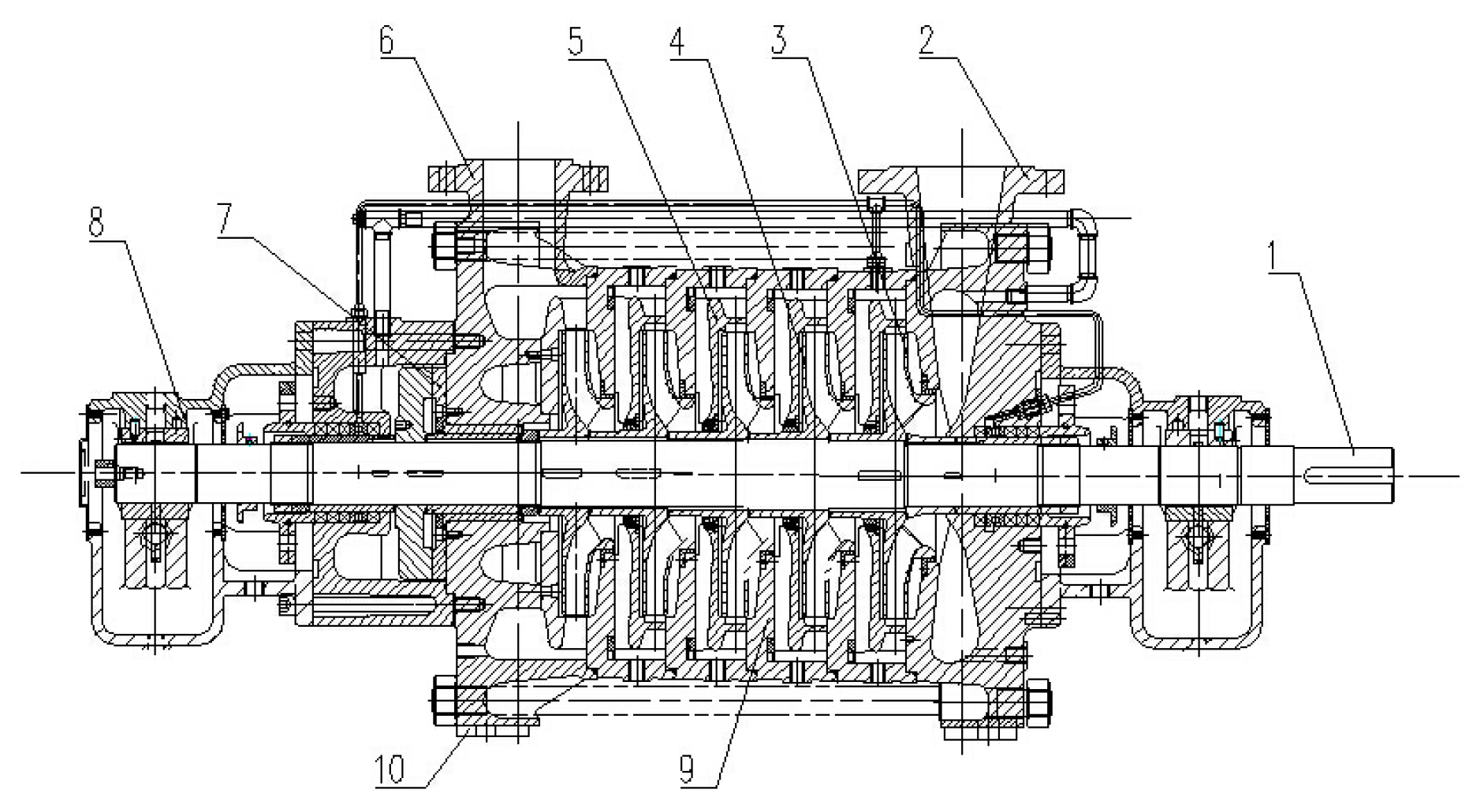Figure 2. The main flow passage components including (a) the impeller and (b) the guide vane.
Figure 2. The main flow passage components including (a) the impeller and (b) the guide vane.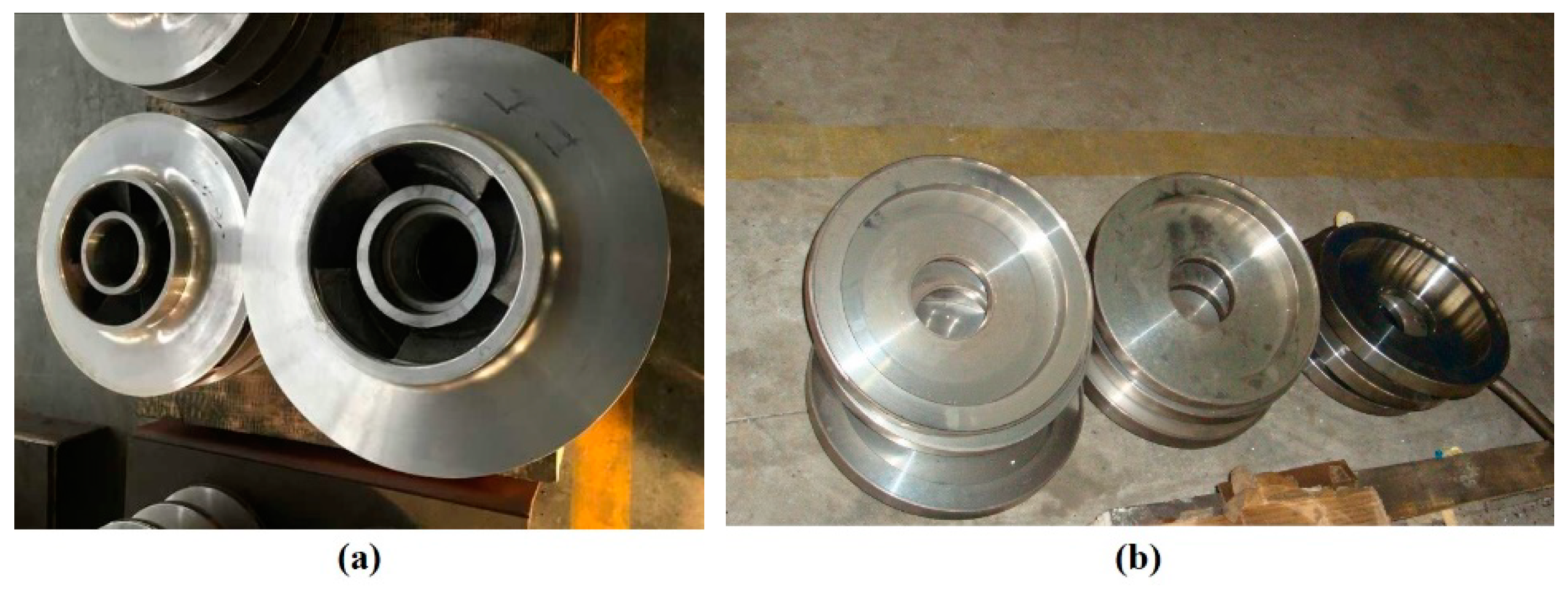Figure 3. Simulation domain.
Figure 3. Simulation domain.Figure 4. The trend of mesh error.
Figure 4. The trend of mesh error.Figure 5. The test system including (a) the pump circulation system and (b) the INV3020C signal acquisition system.
Figure 5. The test system including (a) the pump circulation system and (b) the INV3020C signal acquisition system.Figure 6. Comparison of (a) head and (b) efficiency between numerical simulation and experiments. RNG, Re-normalization Group; SST, Shear Stress Transfer.
Figure 6. Comparison of (a) head and (b) efficiency between numerical simulation and experiments. RNG, Re-normalization Group; SST, Shear Stress Transfer.Figure 7. Comparison of pressure pulsation between simulation and experiment for (a) 0.8 of the design flow rate (Qd), (b) 1.0 Qd, and (c) 1.2 Qd.
Figure 7. Comparison of pressure pulsation between simulation and experiment for (a) 0.8 of the design flow rate (Qd), (b) 1.0 Qd, and (c) 1.2 Qd.Figure 8. The (a) experiment and (b) simulation models of vibration.
Figure 8. The (a) experiment and (b) simulation models of vibration.Figure 9. Dipole source and acoustic boundaries.
Figure 9. Dipole source and acoustic boundaries.Figure 10. The (a) distribution of noise monitoring points and (b) noise experiment scene.
Figure 10. The (a) distribution of noise monitoring points and (b) noise experiment scene.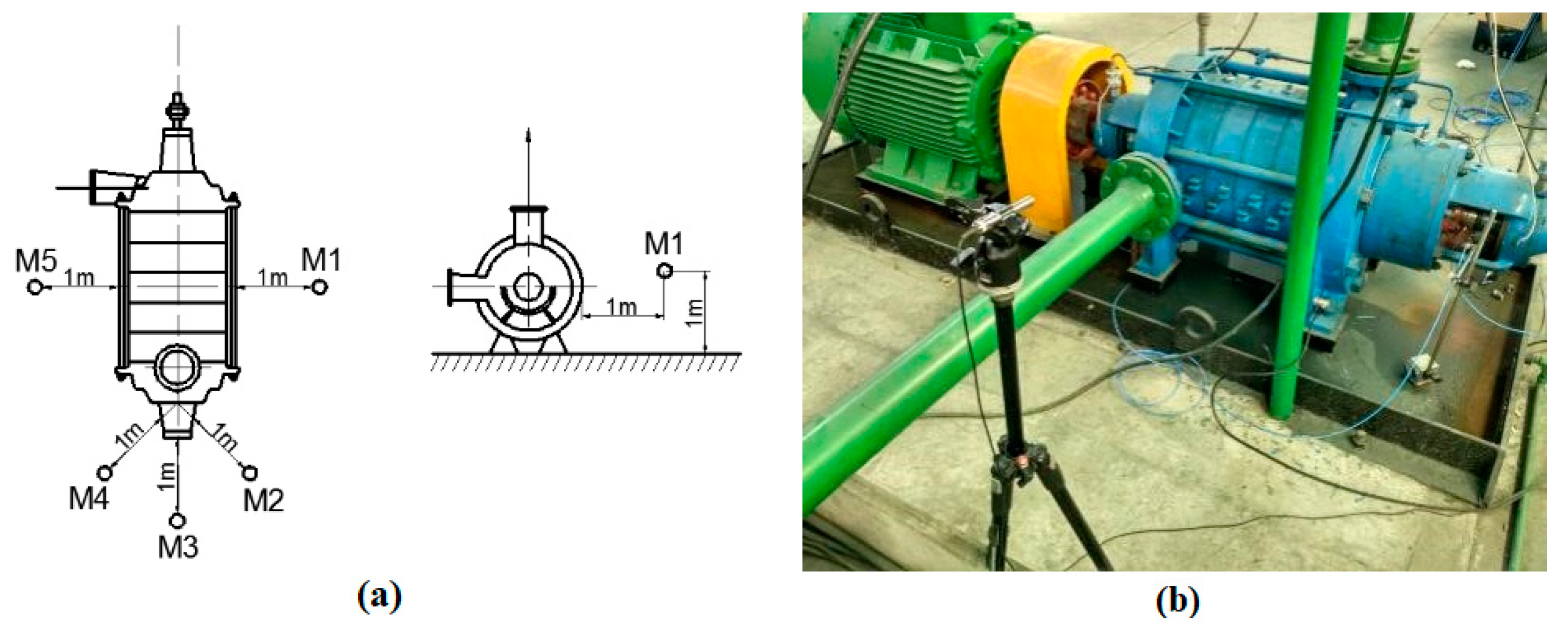Figure 11. Distribution of external noise monitoring points in the simulations.
Figure 11. Distribution of external noise monitoring points in the simulations.Figure 12. Vibration comparison between simulation and experiment. APF, axial passing frequency; BPF1, first blade passing frequency; BPF2, secondary blade passing frequency.
Figure 12. Vibration comparison between simulation and experiment. APF, axial passing frequency; BPF1, first blade passing frequency; BPF2, secondary blade passing frequency.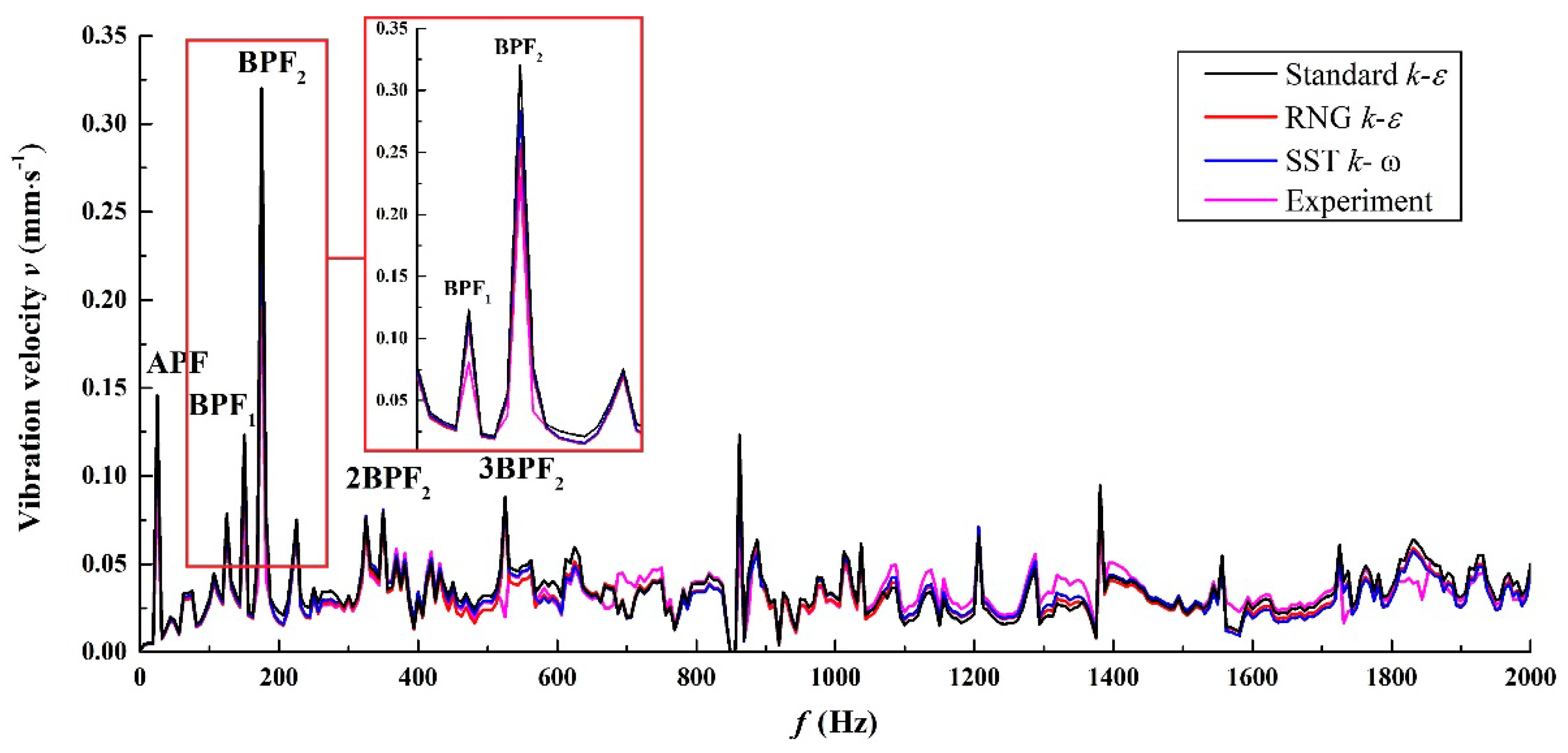Figure 13. Lp at (a) suction and (b) discharge.
Figure 13. Lp at (a) suction and (b) discharge.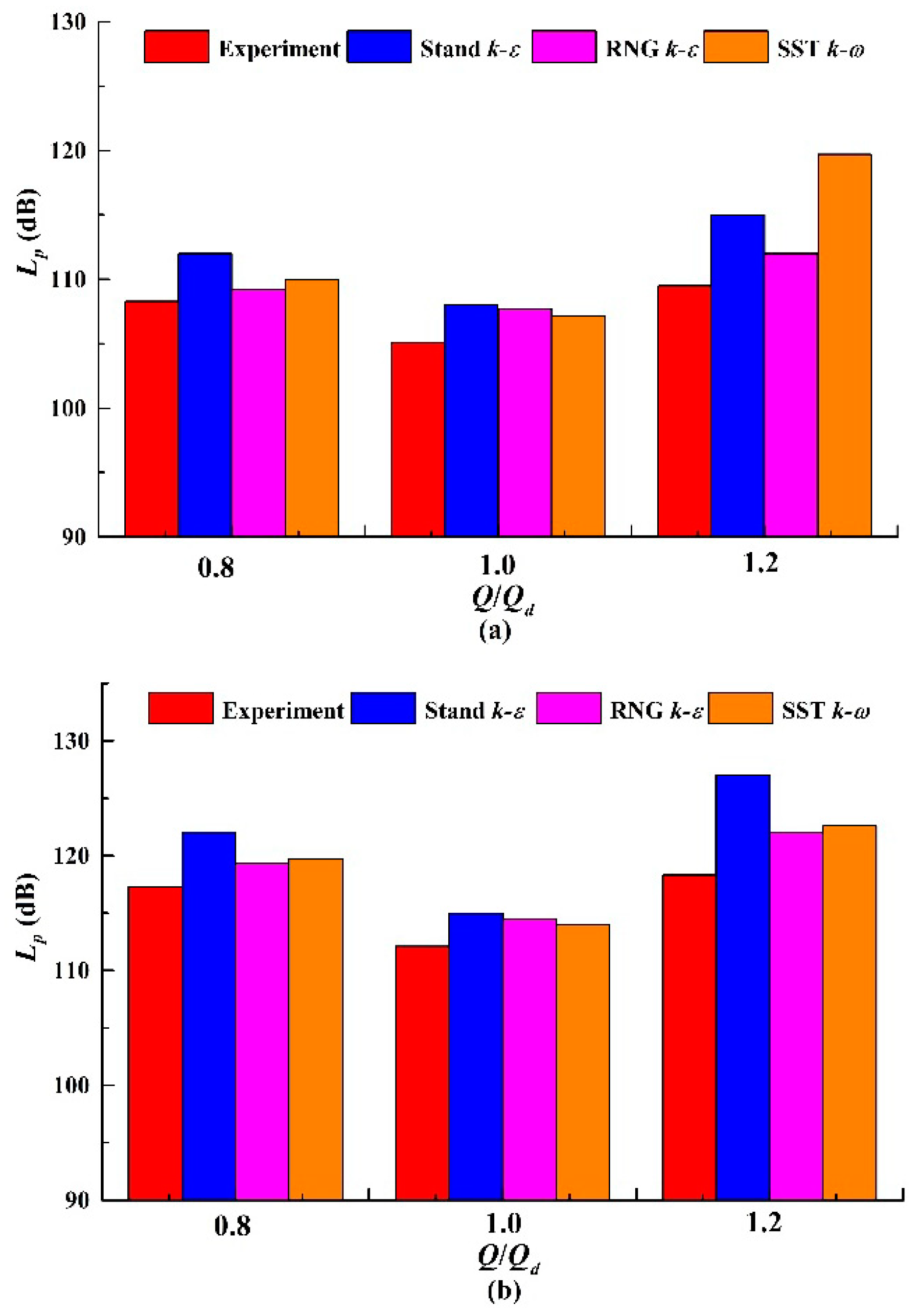Figure 14. Sound pressure level SPL at the suction section for (a) 0.8 Qd, (b) 1.0 Qd, and (c) 1.2 Qd.
Figure 14. Sound pressure level SPL at the suction section for (a) 0.8 Qd, (b) 1.0 Qd, and (c) 1.2 Qd.Figure 15. SPL at the discharge section for (a) 0.8 Qd, (b) 1.0 Qd, and (c) 1.2 Qd.
Figure 15. SPL at the discharge section for (a) 0.8 Qd, (b) 1.0 Qd, and (c) 1.2 Qd.Figure 16. Radiated noise level of simulations and experiment for different monitoring points of (a) M1, (b) M2, (c) M3, (d) M4, and (e) M5.
Figure 16. Radiated noise level of simulations and experiment for different monitoring points of (a) M1, (b) M2, (c) M3, (d) M4, and (e) M5.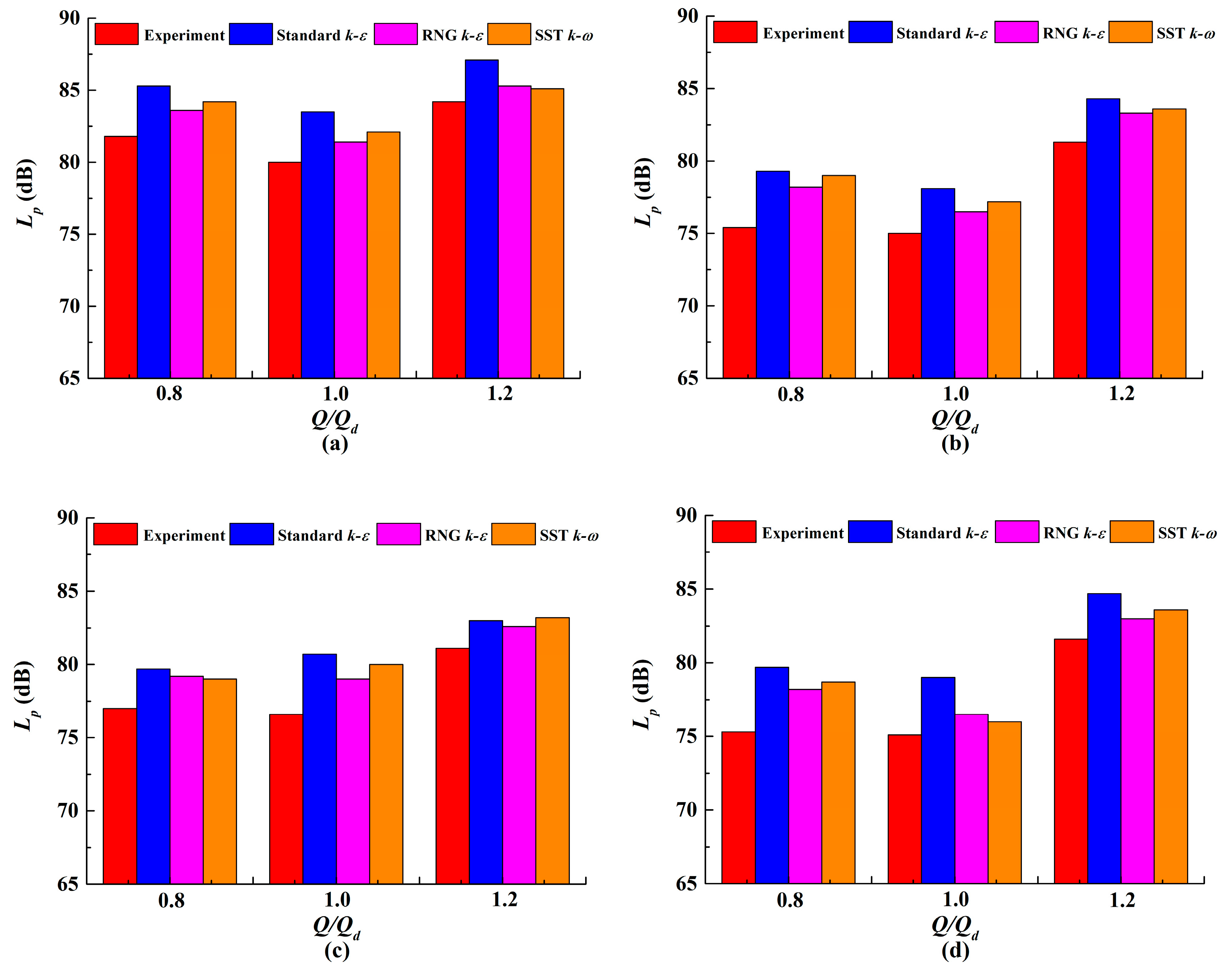Figure 17. Directional distribution of the radiated noise at (a) BPF1 and (b) BPF2.
Figure 17. Directional distribution of the radiated noise at (a) BPF1 and (b) BPF2.Figure 18. Spectrum of the radiated sound power at (a) 0.8 Qd, (b) 1.0 Qd, and (c) 1.2 Qd.
Figure 18. Spectrum of the radiated sound power at (a) 0.8 Qd, (b) 1.0 Qd, and (c) 1.2 Qd.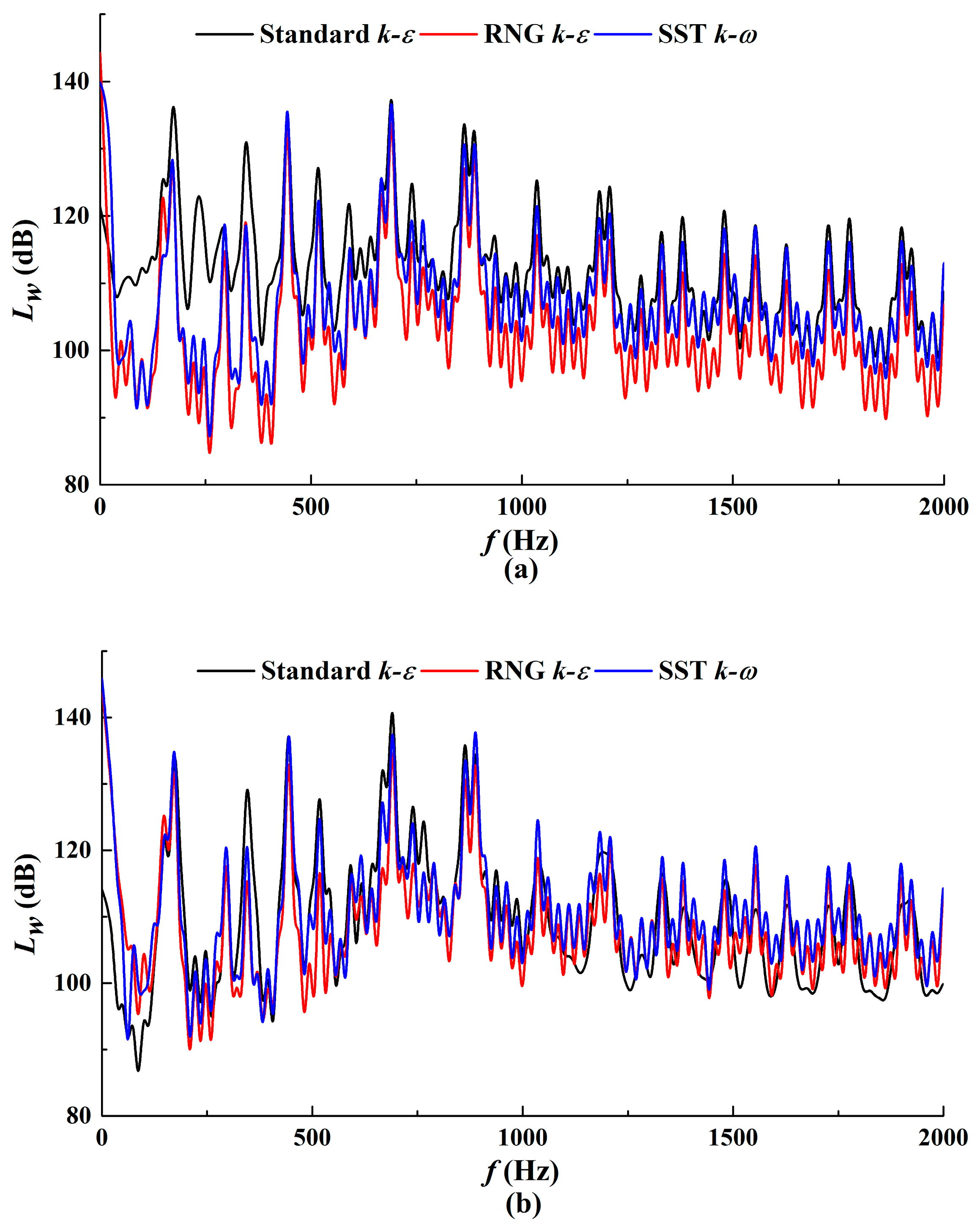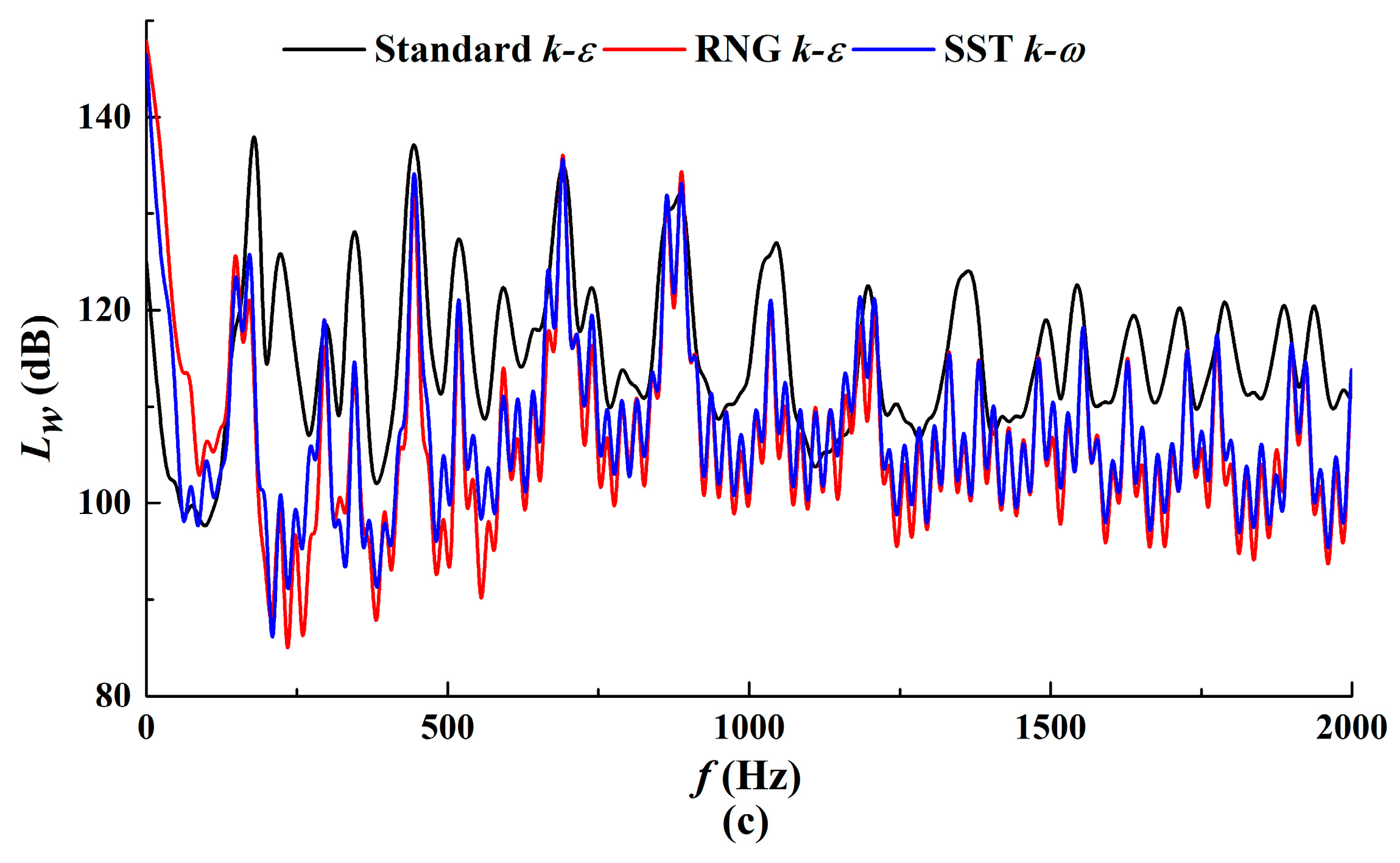Figure 19. Contour of noise band for (a) the standard k-ε, (b) the RNG k-ε, and (c) the SST k-ω model.
Figure 19. Contour of noise band for (a) the standard k-ε, (b) the RNG k-ε, and (c) the SST k-ω model.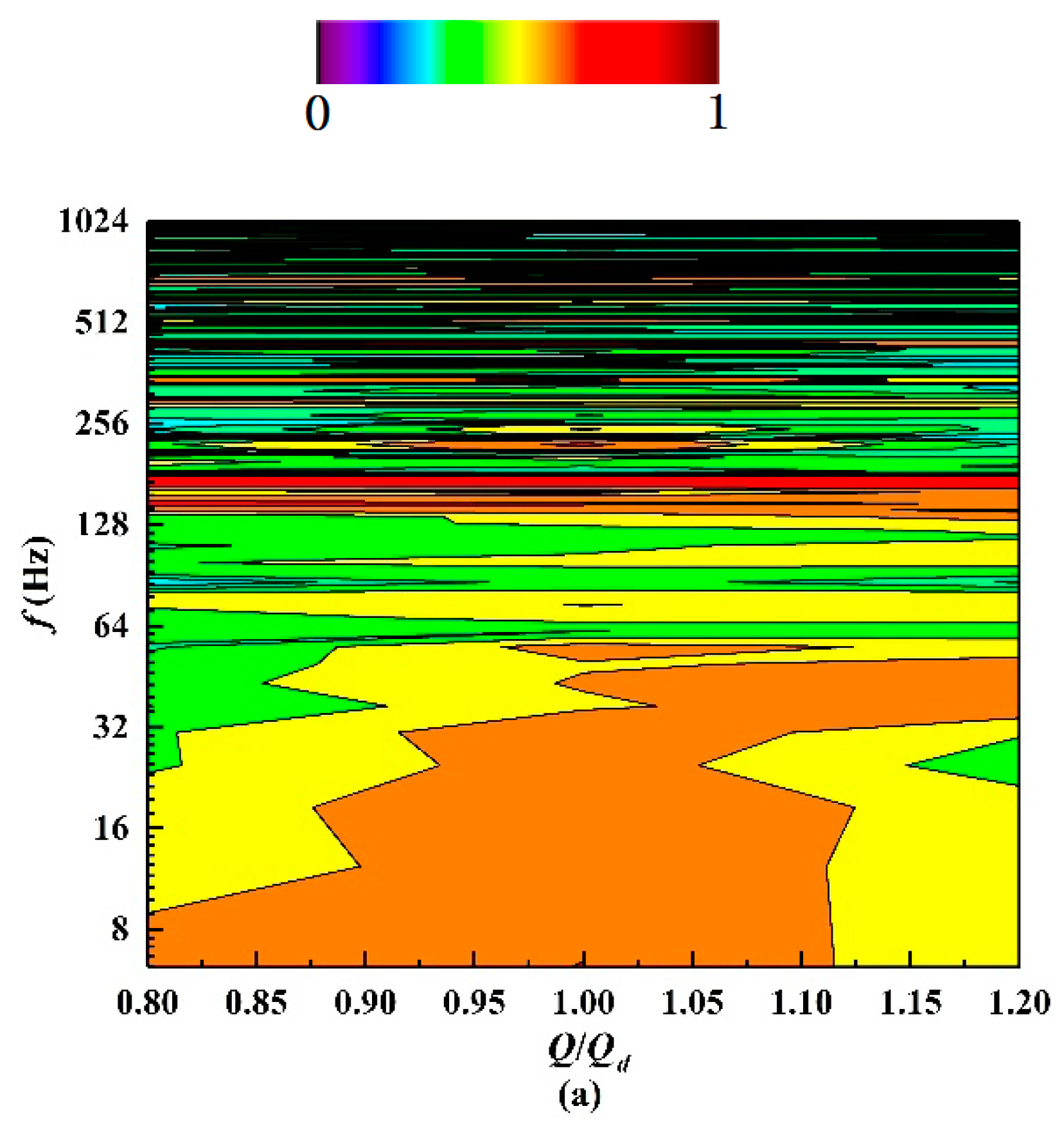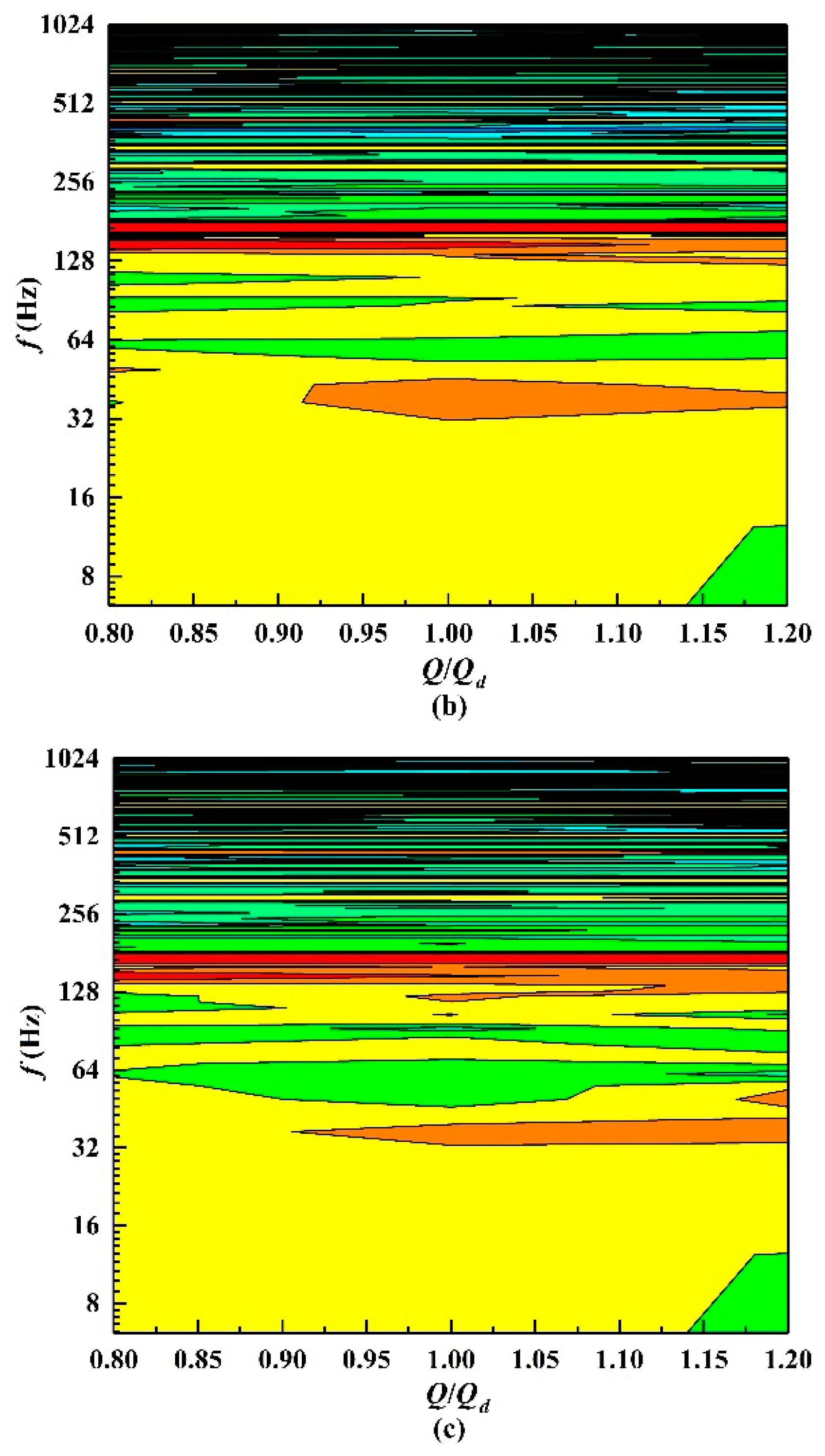Table 1. Main structure parameters.
Table 1. Main structure parameters.
Structure ParametersUnitValues
Inlet diameter of the first-stage impellers Djmm155
Diameter of the first-stage impeller D21mm314
Blade number of the first-stage impeller Z116
Outlet width of the impeller b2mm20.5
Diameter of the subsequent four-stage impellers D22mm314
Blade number of the subsequent four-stage impellers Z217
Inlet diameter of the vaned-diffuser D3mm316
Outlet diameter of the vaned-diffuser D4mm409
Blade numbers of the positive vaned-diffuser Z319
Blade numbers of the negative vaned-diffuser Z4110
Inlet diameter of the pump suction section Dsmm100
Outlet diameter of the pump discharge section Ddmm80
Table 2. Deviation of the sound pressure level Lp of the turbulence models and the experiment.
Table 2. Deviation of the sound pressure level Lp of the turbulence models and the experiment.
DeviationStandard k-εRNG k-εSST k-ω
Suction0.8 Qd3.42%0.83%1.57%
1.0 Qd2.76%2.47%2.00%
1.2 Qd5.02%2.28%9.31%
Discharge0.8 Qd4.01%1.71%2.05%
1.0 Qd2.59%2.14%1.69%
1.2 Qd7.35%3.13%3.63%
Table 3. Deviation of Lp between the turbulence models and the experimental data for the external sound field.
Table 3. Deviation of Lp between the turbulence models and the experimental data for the external sound field.
DeviationStandard k-εRNG k-εSST k-ω
M30.8 Qd3.51%2.86%2.60%
1.0 Qd5.35%3.14%4.44%
1.2 Qd2.34%1.85%2.59%
Back to TopTop